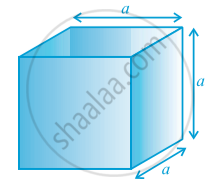Share

# Volume of a Cuboid

#### notes

The measure of this occupied space is called the Volume of the object.
The area of the plane region occupied by each rectangle × height
= Measure of the space occupied by the cuboid
So, we get A × h = V

That is, Volume of a Cuboid = base area × height = length × breadth × height

or l × b × h, where l, b and h are respectively the length, breadth and height of the cuboid.

Note : When we measure the magnitude of the region of a space, that is, the space occupied by a solid, we do so by counting the number of cubes of edge of unit length that can fit into it exactly. Therefore, the unit of measurement of volume is cubic unit.

Again

Volume of a Cube = edge × edge × edge = a^3
where a is the edge of the cube in following fig.So, if a cube has edge of 12 cm ,
Then volume of the cube = 12 × 12 × 12 cm^3
=1728 cm^3.

### Shaalaa.com

Volume of a Cuboid [00:02:27]
S
0%

S